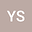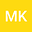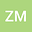A parametric (b, θ)-metric space and some fixed point theorems
•••In this paper, motivated by Kamran et al. [Mathematics, 5(19), (2017), 7 pages] we introduce the notion of parametric $(b, \theta)$-metric space as an extended form of parametric $b$-metric space, which was introduced by Hussain et al.[J. Nonlinear Sci. Appl., 8 (2015), 719–739] and improve the results of Alsulami et al.[Abstract and Applied Analysis, (2014), Article ID 187031, 10 pages] and others in such space. We also apply our result to establish an existence of solution of integral equation.